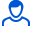Content: teorver_5599.doc (28.50 KB)

Positive responses: 0
Negative responses: 0

Refunds: 05599. x̅=34.3, n=73, σ=1.5. Find the confidence interval for the mathematical expectation a of a normally distributed quantitative attribute X of the general population with reliability γ=0.95, knowing the sample mean x̅в=34.3; sample size n=73 and standard deviation σ=1.5.
Detailed solution. Decorated in Microsoft Word 2003 (Quest decided to use the formula editor)
No feedback yet